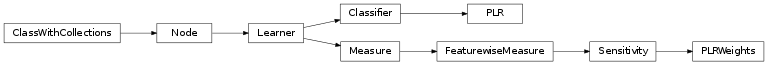# mvpa2.clfs.plr¶

Penalized logistic regression classifier.Functions

Classes

 `PLR`([lm, criterion, reduced, maxiter]) Penalized logistic regression `Classifier`. `PLRWeights`(clf[, force_train]) `Sensitivity` reporting linear weights of PLR

Exceptions

 `PLR`([lm, criterion, reduced, maxiter]) Penalized logistic regression `Classifier`. `PLRWeights`(clf[, force_train]) `Sensitivity` reporting linear weights of PLR